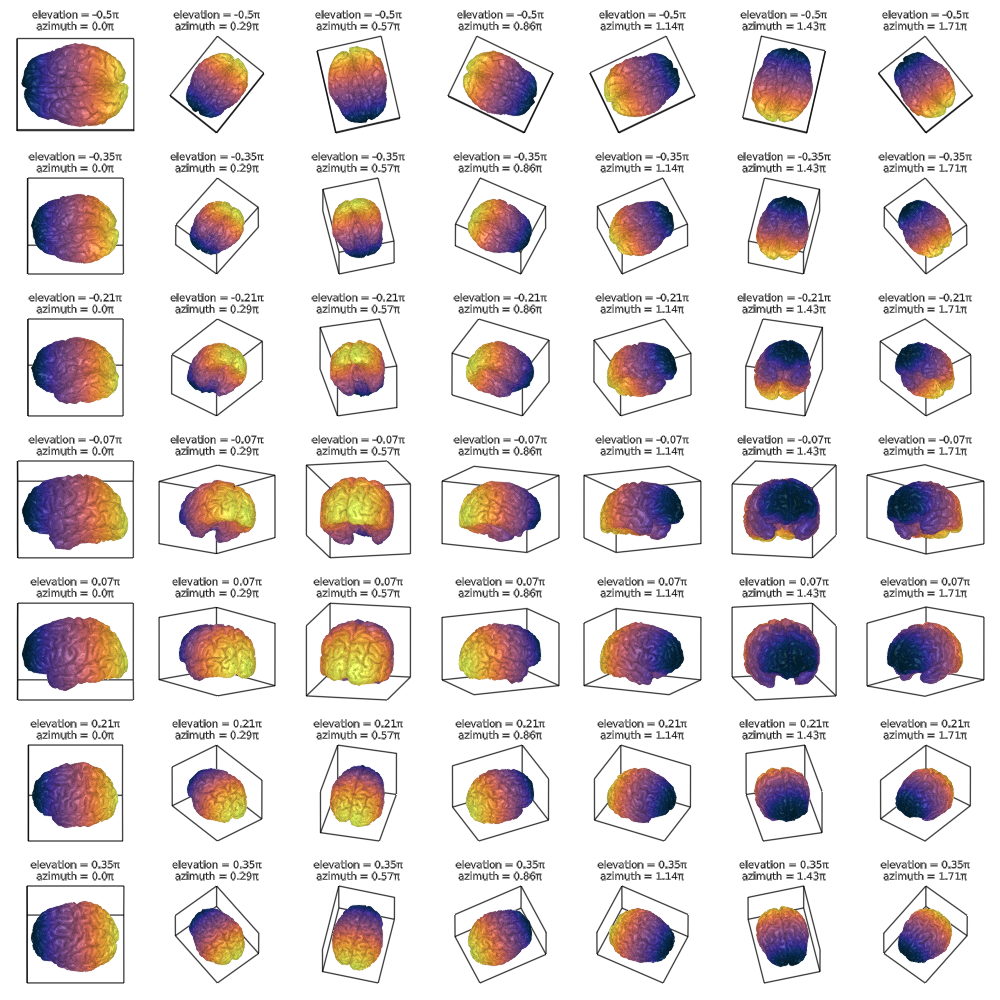# Axis3

## Viewing angles

The two attributes azimuth and elevation control the angles from which the plots are viewed.

using GLMakie
using FileIO

f = Figure(resolution = (1000, 1000), fontsize = 10)

colors = [tri for tri in brain for i in 1:3]

azimuths = LinRange(0, 2pi, 8)[1:7]
elevations = LinRange(-pi/2 + 0.01, pi/2 - 0.01, 8)[1:7]

for (i, elevation) in enumerate(elevations)
for (j, azimuth) in enumerate(azimuths)
ax = Axis3(f[i, j], aspect = :data,
title = "elevation = $(round(elevation/pi, digits = 2))π\nazimuth =$(round(azimuth/pi, digits = 2))π",
elevation = elevation, azimuth = azimuth,
protrusions = (0, 0, 0, 20))

hidedecorations!(ax)
mesh!(brain, color = colors, colormap = :thermal)
end
end

f## Data aspects and view mode

The attributes aspect and viewmode both influence the apparent relative scaling of the three axes.

### aspect

The aspect changes how long each axis is relative to the other two.

If you set it to :data, the axes will be scaled according to their lengths in data space. The visual result is that objects with known real-world dimensions look correct and not squished.

You can also set it to a three-tuple, where each number gives the relative length of that axis vs the others.

using GLMakie
using FileIO

f = Figure(resolution = (1000, 800), fontsize = 14)